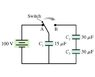# Potential difference in capacitor help

rayhan619

## Homework Statement

Initially, the switch in the figure is in position A and capacitors C_2 and C_3 are uncharged. Then the switch is flipped to position B. (Figure attached)
a) Afterward, what is the charge on C_1 capacitor?
b) Afterward, what is the potential difference across C_1 capacitor?
c) Afterward, what is the charge on C_2 capacitor?
d) Afterward, what is the potential difference across C_2 capacitor?
e) Afterward, what is the charge on C_3 capacitor?
f) Afterward, what is the potential difference across C_3 capacitor?

V= IR

## The Attempt at a Solution

How do I start this problem?

Homework Helper

... (Figure attached)
... How do I start this problem?

This would be a start.

rayhan619

and here is the figure...

#### Attachments

•1.jpg
6.3 KB · Views: 654
Homework Helper

So what do you think is going to happen?

What is the equivalent capacitance of the additional capacitors that are not initially connected?

rayhan619

we know C = Q/V
V = 100 V
C1 = 15*10^-6 F
so Q = C1V = 100 V(15*10^-6 F) = 1.5*10^-3 C

C2 and C3 is in series. so 1/C = (1/C2 + 1/C3) = (1/30*10^-6)+(1/30*10^-6) = 66666.67
so C = 1.5*10^-5

would the Q be same after switching to point b? If yes, then we can figure out V using above equation.

Homework Helper

we know C = Q/V
V = 100 V
C1 = 15*10^-6 F
so Q = C1V = 100 V(15*10^-6 F) = 1.5*10^-3 C

C2 and C3 is in series. so 1/C = (1/C2 + 1/C3) = (1/30*10^-6)+(1/30*10^-6) = 66666.67
so C = 1.5*10^-5

would the Q be same after switching to point b? If yes, then we can figure out V using above equation.

When the connection is broken then there is a fixed charge on the C1. When the switch connects to C2 and C3 then the charge will be shared according to what the equivalent capacitance for all 3 of them.

C2 and C3 = 2*C1

C2 and C3 equivalent C = C1

When you put them in || you have then C1 + C1 = 2 C1

Q = V*C

So with the new C = 2*old C and charge the same, then Vnew = 1/2*Vold

rayhan619

there are like 6 parts of this problem.
so for part a)
C = Q/V
V = 100 V
C1 = 15*10^-6 F
so Q = C1V = 100 V(15*10^-6 F) = 1.5*10^-3 C
and how do I get the potential difference?

Homework Helper

When uncharged capacitors are connected to the charged capacitors the common potential difference is given by V = (C1V1 + o)/(C1 + C2)